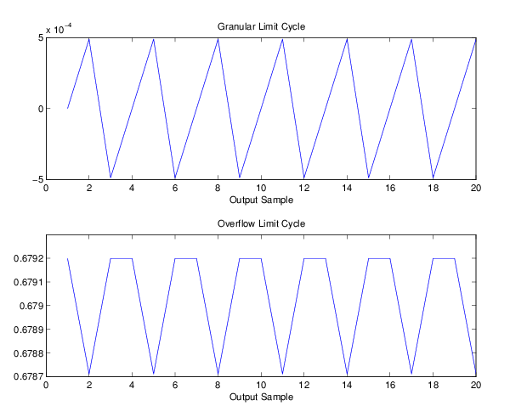Documentation

# limitcycle

Response of single-rate, fixed-point IIR filter

## Syntax

```report = limitcycle(hd) report = limitcycle(hd,ntrials,inputlengthfactor,stopcriterion) ```

## Description

`report = limitcycle(hd)` returns the structure `report` that contains information about how filter `hd` responds to a zero-valued input vector. By default, the input vector has length equal to twice the impulse response length of the filter.

`limitcycle` returns a structure whose elements contain the details about the limit cycle testing. As shown in this table, the report includes the following details.

Output Object Property

Description

`LimitCycleType`

Contains one of the following results:

• `Granular` — indicates that a granular overflow occurred.

• `Overflow` — indicates that an overflow limit cycle occurred.

• `None` — indicates that the test did not find any limit cycles.

`Zi`

Contains the initial condition value(s) that caused the detected limit cycle to occur.

`Output`

Contains the output of the filter in the steady state.

`Trial`

Returns the number of the Monte Carlo trial on which the limit cycle testing stopped. For example, `Trial` = `10` indicates that testing stopped on the tenth Monte Carlo trial.

Using an input vector longer than the filter impulse response ensures that the filter is in steady-state operation during the limit cycle testing. `limitcycle` ignores output that occurs before the filter reaches the steady state. For example, if the filter impulse length is 500 samples, `limitcycle` ignores the filter output from the first 500 input samples.

To perform limit cycle testing on your IIR filter, you must set the filter `Arithmetic` property to `fixed` and `hd` must be a fixed-point IIR filter of one of the following forms:

• `df1` — direct-form I

• `df1t` — direct-form I transposed

• `df1sos` — direct-form I with second-order sections

• `df1tsos` — direct-form I transposed with second-order sections

• `df2` — direct-form II

• `df2t` — direct-form II transposed

• `df2sos` — direct-form II with second-order sections

• `df2tsos` — direct-form II transposed with second-order sections

When you use `limitcycle` without optional input arguments, the default settings are

• Run 20 Monte Carlo trials

• Use an input vector twice the length of the filter impulse response

• Stop testing if the simulation process encounters either a granular or overflow limit cycle

To determine the length of the filter impulse response, use `impzlength`:

`impzlength(hd)`

During limit cycle testing, if the simulation runs reveal both overflow and granular limit cycles, the overflow limit cycle takes precedence and is the limit cycle that appears in the report.

Each time you run `limitcycle`, it uses a different sequence of random initial conditions, so the results can differ from run to run.

Each Monte Carlo trial uses a new set of randomly determined initial states for the filter. Test processing stops when `limitcycle` detects a zero-input limit cycle in filter `hd`.

`report = limitcycle(hd,ntrials,inputlengthfactor,stopcriterion)` returns a report of how filter `hd` responds to a zero-valued input vector, using the following optional input arguments:

• `ntrials` — Number of Monte Carlo trials (default is 20).

• `inputlengthfactor` — integer factor used to calculate the length of the input vector. The length of the input vector comes from (`impzlength(hd)` * `inputlengthfactor`), where `inputlengthfactor` = 2 is the default value.

• `stopcriterion` — the criterion for stopping the Monte Carlo trial processing. `stopcriterion` can be set to `either` (the default), `granular`, `overflow`. This table describes the results of each stop criterion.

stopcriterion Setting

Description

`either`

Stop the Monte Carlo trials when `limitcycle` detects either a granular or overflow limit cycle.

`granular`

Stop the Monte Carlo trials when `limitcycle` detects a granular limit cycle.

`overflow`

Stop the Monte Carlo trials when `limitcycle` detects an overflow limit cycle.

### Note

An important feature is that if you specify a specific limit cycle stop criterion, such as `overflow`, the Monte Carlo trials do not stop when testing encounters a granular limit cycle. You receive a warning that no `overflow` limit cycle occurred, but consider that a `granular` limit cycle might have occurred.

## Examples

In this example, there is a region of initial conditions in which no limit cycles occur and a region where they do. If no limit cycles are detected before the Monte Carlo trials are over, the state sequence converges to zero. When a limit cycle is found, the states do not end at zero. Each time you run this example, it uses a different sequence of random initial conditions, so the plot you get can differ from the one displayed in the following figure.

```s = [1 0 0 1 0.9606 0.9849]; hd = dfilt.df2sos(s); hd.arithmetic = 'fixed'; greport = limitcycle(hd,20,2,'granular') oreport = limitcycle(hd,20,2,'overflow') figure, subplot(211),plot(greport.Output(1:20)), title('Granular Limit Cycle'); subplot(212),plot(oreport.Output(1:20)), title('Overflow Limit Cycle'); greport = LimitCycle: 'granular' Zi: [2x1 double] Output: [1303x1 embedded.fi] Trial: 1 oreport = LimitCycle: 'overflow' Zi: [2x1 double] Output: [1303x1 embedded.fi] Trial: 2```

The plots shown in this figure present both limit cycle types — the first displays the small amplitude granular limit cycle, the second the larger amplitude overflow limit cycle.As you see from the plots, and as is generally true, overflow limit cycles are much greater magnitude than granular limit cycles. This is why `limitcycle` favors overflow limit cycle detection and reporting.